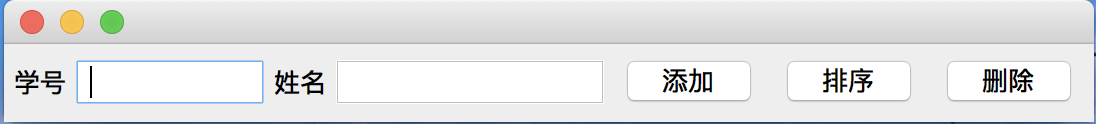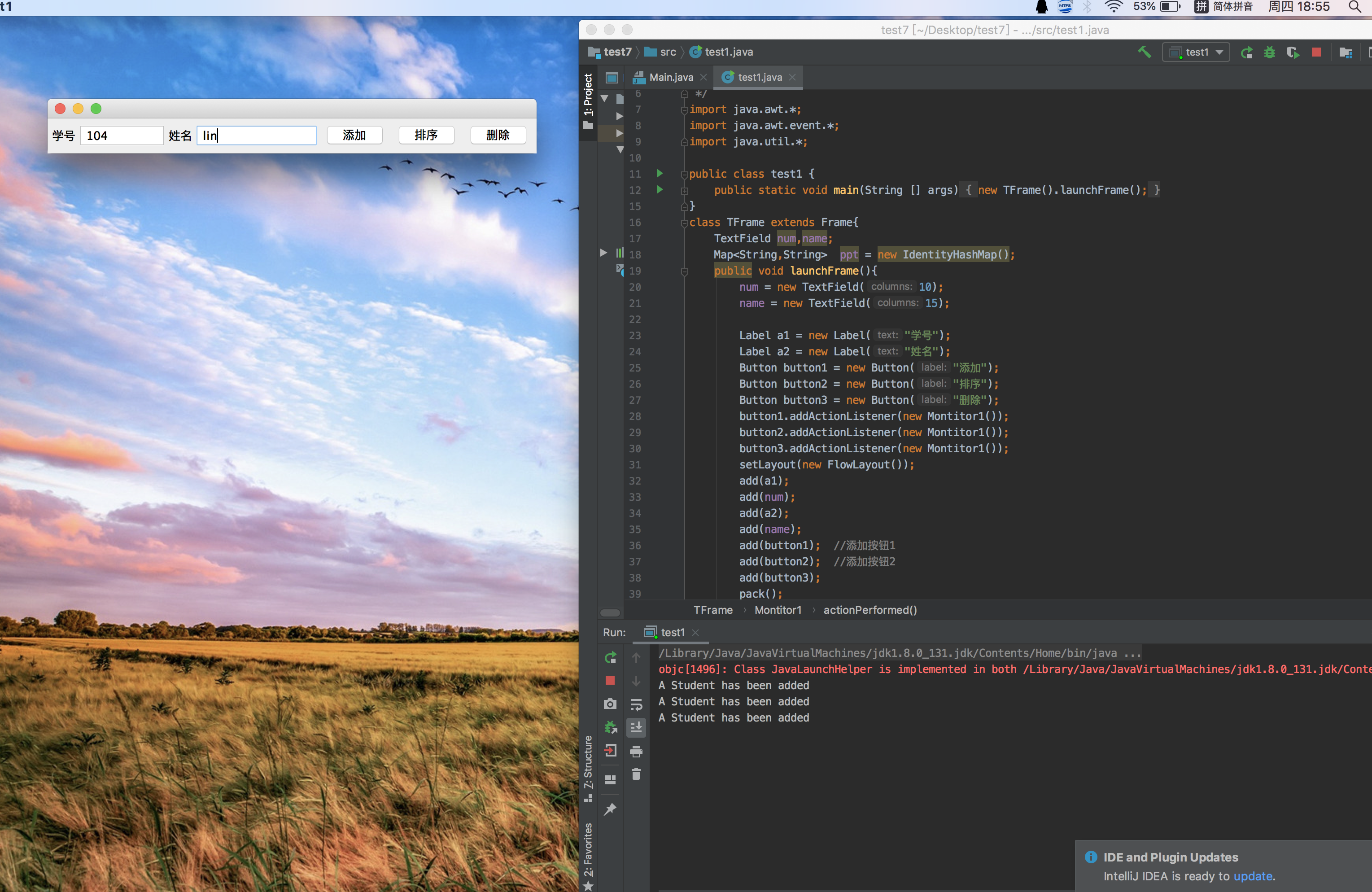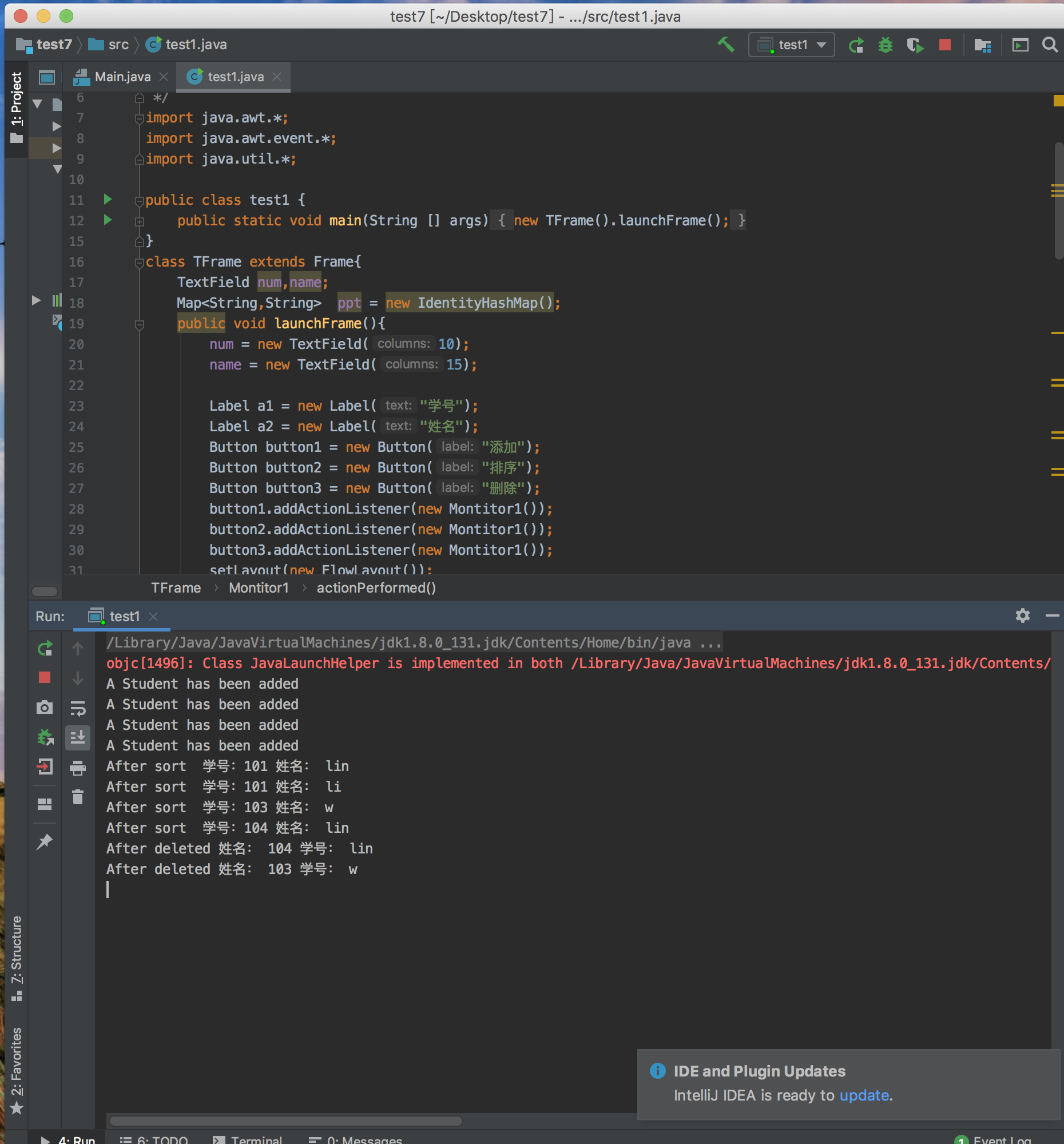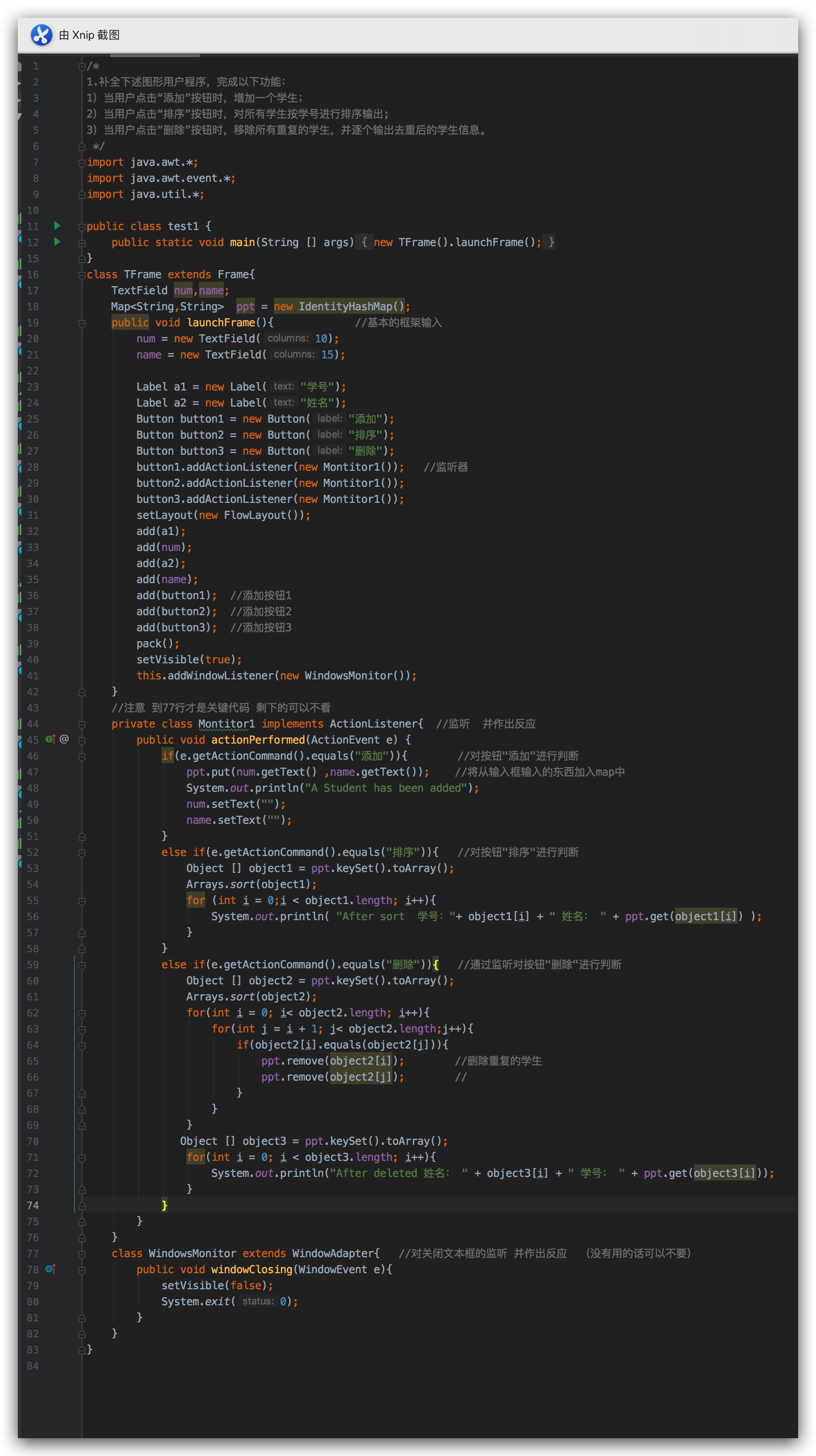• 总分排序3.数学成绩排序4.语文成绩排序5.计算机成绩排序6.单得成绩均大于85分的学生7.将结果输出至文本文件0.退出系统package test_java;import java.util.ArrayList;import java.util.Random;import java....

要求：

1.请输入5个学生

2.按总分排序

3.按数学成绩排序

4.按语文成绩排序

5.按计算机成绩排序

6.单得成绩均大于85分的学生

7.将结果输出至文本文件

0.退出系统

package test_java;

import java.util.ArrayList;

import java.util.Random;

import java.util.Scanner;

import java.io.*;

@SuppressWarnings("serial")

{

private int ID;

private String Name;

private int Math;

private int Chinese;

private int Computer;

private int Total;

{

ID=0;

Name="";

Math=0;Chinese=0;Computer=0;

Total=0;

}

void setGrade(int id,String name,int math,int chinese,int computer)

{

ID=id;

Name=name;

Math=math;

Chinese=chinese;

Computer=computer;

Total=Math+Chinese+Computer;

}

int getID()

{

return ID;

}

String getName()

{

return Name;

}

int getMath()

{

return Math;

}

int getChinese()

{

return Chinese;

}

int getComputer()

{

return Computer;

}

int getTotal()

{

}

void show()

{

System.out.print(ID);System.out.print(‘\t‘);

System.out.print(Name);System.out.print(‘\t‘);

System.out.print(Math);System.out.print(‘\t‘);

System.out.print(Chinese);System.out.print(‘\t‘);

System.out.print(Computer);System.out.print(‘\t‘);

System.out.print(Total);System.out.println(‘\t‘);

}

}

interface Ifun

{

abstract int quickpatition(Grade r[],int low,int high);

abstract void chinese_sort(Grade stu[],int low,int high);

}

abstract class chinesesort implements Ifun

{

{

int i,j;

if(low

{

i=low;

j=high;

w=r[low];

while(i

{

while((r[j].getChinese()>w.getChinese()) && (i

j--;

if(i

r[i++]=r[j];

while((r[i].getChinese()<=w.getChinese()) && (i

i++;

if(i

r[j--]=r[i];

}

r[i]=w;

}

return low;

}

public void chinese_sort(Grade stu[],int low,int high)

{

if(low

{

int temp=quickpartition(stu,low,high);

chinese_sort(stu,low,temp-1);

chinese_sort(stu,temp+1,high);

}

}

}

//冒泡排序类

class bubble

{

}

public class java1

{

//退出菜单

static void _exit()

{

System.out.println("************************************************");

System.out.println("");

System.out.println("     欢迎使用本系统");

System.out.println("");

System.out.println("         再见！");

System.out.println("");

System.out.println("************************************************");

}

//表头信息

static void table()

{

System.out.print("学号");System.out.print(‘\t‘);

System.out.print("姓名");System.out.print(‘\t‘);

System.out.print("数学");System.out.print(‘\t‘);

System.out.print("语文");System.out.print(‘\t‘);

System.out.print("计算机");System.out.print(‘\t‘);

System.out.print("总分");System.out.println(‘\t‘);

}

//主菜单

{

System.out.println("************************************************");

System.out.println("     1.请输入5个学生");

System.out.println("     2.按总分排序");

System.out.println("     3.按数学成绩排序");

System.out.println("     4.按语文成绩排序");

System.out.println("     5.按计算机成绩排序");

System.out.println("     6.单得成绩均大于85分的学生");

System.out.println("     7.将结果输出至文本文件");

System.out.println("     0.退出系统");

System.out.println("************************************************");

}

//按总成绩冒泡排序

{

int i,j;

for(i=0;i

{

for(j=0;j

{

if(stu[j].getTotal()

{

temp=stu[j];

stu[j]=stu[j+1];

stu[j+1]=temp;

}

}

}

}

//按语文成绩--快速排序

static int quickpartition(Grade r[],int low,int high)

{

int i,j;

if(low

{

i=low;

j=high;

w=r[low];

while(i

{

while((r[j].getChinese()>w.getChinese()) && (i

j--;

if(i

r[i++]=r[j];

while((r[i].getChinese()<=w.getChinese()) && (i

i++;

if(i

r[j--]=r[i];

}

r[i]=w;

}

return low;

}

static void chinese_sort(Grade stu[],int low,int high)

{

if(low

{

int temp=quickpartition(stu,low,high);

chinese_sort(stu,low,temp-1);

chinese_sort(stu,temp+1,high);

}

}

//按数学成绩--直接选择排序

{

System.out.println("按数学成绩由小到大排序");

int min,j;

for(int i=0;i

{

min=i;

for(j=i;j

{

if(stu[min].getMath()>stu[j].getMath())

{

min=j;

}

}

if(min!=j)

{

temp=stu[i];

stu[i]=stu[min];

stu[min]=temp;

}

}

}

//按计算机成绩--直接插入排序

{

System.out.println("按计算机成绩由小到大排序");

int i,j;

for(i=1;i

{

temp=stu[i];

j=i-1;

while(j>0 && temp.getComputer() < stu[j].getComputer())

{

stu[j+1]=stu[j];

j--;

}

stu[j+1]=temp;

}

}

public static void main(String[] args) throws Exception

{

int num;

int i;

final int N=5;

int id;

String name;

int math;

int chinese;

int computer;

//ArrayList student=new ArrayList();

while(true)

{

System.out.print("请选择：");

switch(num)

{

case 1:

System.out.println("请输入5个学生的情况：");

for(i=0;i

{

//System.out.println("请输入学生的学号:");

//System.out.println("请输入学生的姓名:");

//System.out.println("请输入数学成绩:");

//System.out.println("请输入语文成绩:");

//System.out.println("请输入计算机成绩:");

}

table();//获得表头信息

//按输入的顺序显示5个学生的信息

for(i=0;i

{

stu[i].show();

}

break;

//break;

case 2:

System.out.println("按总分冒泡排序");

bubble_sort(stu,N);

table();

for(i=0;i

{

stu[i].show();

}

break;

case 3:

System.out.println("按语文成绩快速排序:");

chinese_sort(stu,0,N-1);

table();

for(i=0;i

{

stu[i].show();

}

break;

case 4:

System.out.println("按数学成绩直接选择排序:");

math_sort(stu,N);

table();

for(i=0;i

{

stu[i].show();

}

break;

case 5:

System.out.println("按计算机成绩直接插入排序:");

computer_sort(stu,N);

table();

for(i=0;i

{

stu[i].show();

}

break;

case 6:

System.out.println("单科成绩大于85分以上的学生：");

for(i=0;i

{

if(stu[i].getMath()>85 &&

stu[i].getChinese()>85 &&

stu[i].getComputer()>85)

stu[i].show();

}

break;

case 7:

System.out.println("将文件结果输出至文本文件");

for(i=0;i

{

System.out.println("stu的对象id:"+stu[i].getID());

System.out.println("stu的对象id:"+stu[i].getName());

System.out.println("stu的对象id:"+stu[i].getMath());

}

FileOutputStream fos=new FileOutputStream("c:\\aa\\file1.txt");

ObjectOutputStream oos=new ObjectOutputStream(fos);

oos.writeObject(stu);

/*

try

{

ObjectOutputStream os=new ObjectOutputStream(new FileOutputStream("c:/file1.txt"));

os.writeObject(stu);

os.flush();

os.close();

}catch(FileNotFoundException e)

{

e.printStackTrace();

}catch(IOException e)

{

e.printStackTrace();

}

*/

//Random rand=new Random();

/*

FileOutputStream out=new FileOutputStream("/Users/sx_dqbsina.com/file2.txt");

ObjectOutputStream oos=new ObjectOutputStream(out);

oos.writeObject(stu);

oos.flush();

oos.close();

//byte b[]=stu

FileWriter writer=new FileWriter("/Users/sx_dqbsina.com/file2.txt");

//writer.write(stu);

//BufferedWriter output;

//try {

output = new BufferedWriter(new FileWriter("/Users/sx_dqbsina.com/file2.txt"));

//} catch (IOException e) {

// TODO Auto-generated catch block

//e.printStackTrace();

}

BufferedWriter bw=new BufferedWriter(writer);

int []num1=new int;

for(i=0;i

{

num1[i]=20+rand.nextInt(9979);

output.write(num1[i]+"");

output.newLine();

}

//output.close();

*/

break;

case 0:

_exit();

System.exit(1);

//System.out.println("0");

break;

default:

System.out.println("没有此项目！");

//break;

}

}

//System.out.println(z);

}

}

展开全文• 定义学生信息结构体，录入学生信息，根据学生学号顺序进行排序。 struct student 定义学生信息学生信息中Score sc 为一个结构体类型的变量，存放学生的成绩信息； input(),disp()函数输入输出学生信息；sort()...

定义学生信息结构体，录入学生信息，根据学生的学号顺序进行排序。
struct student 定义学生信息，学生信息中Score sc 为一个结构体类型的变量，存放学生的成绩信息；
input(),disp()函数输入输出学生信息；sort()通过学号的大小进行排序；程序中运用了选择法排序；若想对其他信息排序均可以类似的方法进行。

#include<iostream>
using namespace std;

struct Score   //存成绩
{
int math;
int English;
int computer;
};
struct student
{
int num;
char name;
Score sc;
};
void input(student *stu, int n);
void disp(student *p, int n);
void sort(student *sarr, int n);

int main()
{
student stu;
input(stu, 4);
sort(stu, 4);
disp(stu, 4);
return 0;
}
void input(student *stu, int n)
{
cout << "input the student's imformations:" << endl;
for (int i = 0; i < n; i++)
{
cin >> stu[i].num >> stu[i].name >> stu[i].sc.math >> stu[i].sc.English >> stu[i].sc.computer;
}
}
void disp(student *stu, int n)
{
cout << "output the student's informations" << endl;
for (int i = 0; i < n;i++)
{
cout << stu[i].num << ' ' << stu[i].name << ' ' << stu[i].sc.math << ' '
<< stu[i].sc.English << ' ' << stu[i].sc.computer << ' ' << endl;
}
}
void sort(student *stu, int n)
{
int k;
student tmp;   //student类型的tmp变量
for (int i = 0; i < n;i++)
{
k = i;
for (int j = i + 1; j < n; j++)
{
if (stu[j].num<stu[i].num)
{
k = j;
}
if (k != i)
{
tmp = stu[k];
stu[k] = stu[i];
stu[i] = tmp;
}
}
}
}

程序结果测试成功，可直接运行。

展开全文选择法排序 结构体
• 最终 输入并排序结果如下图 （如当输入的有删除情况时 点击删除 将重复的数据删除 并输出删除后的结果） ： ** 代码如下： ** 这是代码截屏 嘻嘻???? 下面是源代码： /* 1.补全下述图形用户程序，完成以下功能： 1）...

解释如下

运行窗口界面如下：向其中输入点击添加 显示 ：A student has been added最终 输入并排序结果如下图 （如当输入的有删除情况时 点击删除 将重复的数据删除 并输出删除后的结果） ：**

代码如下：

**
这是代码截屏 嘻嘻😁下面是源代码

/*
1.补全下述图形用户程序，完成以下功能：
1）当用户点击“添加”按钮时，增加一个学生；
2）当用户点击“排序”按钮时，对所有学生按学号进行排序输出;
3）当用户点击“删除”按钮时，移除所有重复的学生，并逐个输出去重后的学生信息。
*/

import java.awt.*;
import java.awt.event.*;
import java.util.*;

public class test1 {
public static void main(String [] args){
new TFrame().launchFrame();
}
}
class TFrame extends Frame{
TextField num,name;
Map<String,String>  ppt = new IdentityHashMap();
public void launchFrame(){             //基本的框架输入
num = new TextField(10);
name = new TextField(15);

Label a1 = new Label("学号");
Label a2 = new Label("姓名");
Button button1 = new Button("添加");
Button button2 = new Button("排序");
Button button3 = new Button("删除");
setLayout(new FlowLayout());
pack();
setVisible(true);
}
//注意 到77行才是关键代码 剩下的可以不看
private class Montitor1 implements ActionListener{  //监听  并作出反应
public void actionPerformed(ActionEvent e) {
if(e.getActionCommand().equals("添加")){        //对按钮"添加"进行判断
ppt.put(num.getText() ,name.getText());    //将从输入框输入的东西加入map中
num.setText("");
name.setText("");
}
else if(e.getActionCommand().equals("排序")){   //对按钮"排序"进行判断
Object [] object1 = ppt.keySet().toArray();
Arrays.sort(object1);
for (int i = 0;i < object1.length; i++){
System.out.println( "After sort  学号："+ object1[i] + " 姓名： " + ppt.get(object1[i]) );
}
}
else if(e.getActionCommand().equals("删除")){   //通过监听对按钮"删除"进行判断
Object [] object2 = ppt.keySet().toArray();
Arrays.sort(object2);
for(int i = 0; i< object2.length; i++){
for(int j = i + 1; j< object2.length;j++){
if(object2[i].equals(object2[j])){
ppt.remove(object2[i]);        //删除重复的学生
ppt.remove(object2[j]);        //
}
}
}
Object [] object3 = ppt.keySet().toArray();
for(int i = 0; i < object3.length; i++){
System.out.println("After deleted 姓名： " + object3[i] + " 学号： " + ppt.get(object3[i]));
}
}
}
}
class WindowsMonitor extends WindowAdapter{   //对关闭文本框的监听 并作出反应  （没有用的话可以不要）
public void windowClosing(WindowEvent e){
setVisible(false);
System.exit(0);
}
}
}

谢谢借鉴 有错的地方 或者更好的方法记得戳我

展开全文java GUI 监听 map
• 先确定出学生人数a，然后利用for循环循环a次，以输入a个学生信息，同时将不同类型信息分开保存至对应列表，最后再将几个列表转化为一个字典，再对字典排序。 实验代码 a=int(input("请输入学生人数：")) num_list=[]...

算法思想

先确定出学生人数a，然后利用for循环循环a次，以输入a个学生信息，同时将不同类型信息分开保存至对应列表，最后再将几个列表转化为一个字典，再对字典排序。

实验代码

a=int(input("请输入学生人数："))
num_list=[]
name_list=[]
d={}
for i in range(a):
num=input("学号为：")
name=input("姓名为：")
print("\n")
num_list.append(num)
name_list.append(name)

d=dict(zip(num_list,name_list))
new_d=dict(sorted(d.items(),key=lambda x:x))
print(new_d)

new_d=dict(sorted(d.items(),key=lambda x:x)) 的解读：
d.items()实际上是将d转换为可迭代对象，将字典元素转化为了列表。key参数对应的lambda表达式的意思则是选取元组中的第一个元素作为比较参数，也就是key值作为比较对象，若将改为key=lambda x:x则是选取第二个元素作为比较对象，也就是values值作为比较对象。

展开全文python
• 怎样用python进行年龄分段并且分享和排序？小编努力地向远方，站在高处，曾经是为了让你一眼看见小编，后来为了可以看见更多的人。，怎样用最简单的Python语言将具体的年纪进行分段并且统计各年龄段def input(): ...
• 编写一个学生信息排序程序。要求：1、可随时输入n个学生信息和成绩（n不设置上限）。2、学生信息包括：学号、姓名、性别、专业、学院；三门课程成绩。3、为用户提供一个排序选择列表，使得用户能够按照上述所列...c语言 链表 排序算法 指针 内存管理
• 在写学生成绩分析时，会给学生一个排名，但一般成绩录入时，都是无序的，如果我们用链表存储信息，那么构造的数据结构中肯定还有其他的信息，如姓名，学号等，所以我们得根据成绩进行链表节点的交换。 1. 从文件中...
• 要求： 用数据文件保存学生基本信息(学号 姓名 课程1 课程2 课程3…) ,利用菜单可以循环实现各个功能。 进一步要求*： (1) 能够实现增加、删除、修改功能； (2) 能够实现统计功能等。 思路简介： 要采用模块化思想，...C语言 学生管理系统
• 数据结构程序代码 利用C++ 将多个（自定义）学生成绩信息输入 总成绩排序输出
• 主要为大家详细介绍了java实现学生成绩录入系统，具有一定的参考价值，感兴趣的小伙伴们可以参考一下
• 初始化10名学生的基本信息，包括学号、姓名、三科(高数、英语、程序设计)成绩，分别计算每名学生的总分，并个人总分对学生降序排序，输出排序后的学生信息编写程序，初始化10名学生的基本信息，包括学号、姓名、...
• 写一个程序，用来存储学生学号和成绩，并分数从低到高排序。请使用链表实现。 例如用户输入：1,90<回车>、2,100<回车>、3,80<回车>、OK<回车>。输出则是：3=80<换行>、1=90<...链表 c语言
• } /****** 函数功能：添加学生信息学号判断该学生是否已存在： 不存在，添加 存在，结束 ******/ void Add(void) { printf("请输入要添加的学号：\n"); int num,i; scanf("%d",&num); for(i=0;i { if(num == stu...c语言
• ①实验要求：键盘录入学生的姓名和分数，并按照分数的升序输出学生信息。  思路：1.创建Studen类，创建name,score,以及涉及到方法。  2.创建StudentTest类，对学生信息进行键入。  3.关于对象属性的比较。这里...
• '''学生信息管理系统：1、采用本地txt文件存储学生信息2、包括学号、姓名、英语成绩、python成绩、java成绩3、实现增、删、改、查、排序、计算总人数、显示全部、退出系统，功能'''filename="students.txt" # 定义...
• # 1、由用户输入学生学号与姓名，数据用字典存储，最终输出学生信息按学号由小到大显示）# 创建字典students = {}# 用户输入student = input("请输入学号：")ID = input("请输入你的姓名:")if not(student is None...
• 键盘录入5个学生信息（姓名，语文成绩，数学成绩，英语成绩），按照总分从高到低输出到控制台 分析： 1，定义一个学生类 * 成员变量：姓名，语文成绩，数学成绩，英语成绩 * 成员方法：空参构造，有参构造；有...
• #include <stdio.h> #include<string.h> #define N 2 void main() { int *q,*r,i,j,t,m,v;... printf("请输入第%d个人姓名、学号、成绩:",i+1); scanf("%s%d%d",str[i],&b[i],&c[i]);c语言
• 该系统模拟一个简单的学生管理系统，要求对文件中所存储的学生数据进行各种 常规操作，如：排序、查找、计算、显示等功能。通过此课题，熟练掌握文件、数组、结构 体的各种操作，在程序设计中体现一定的算法思想，...
• 首先是对整体函数所需要的功能进行子函数的分类，让信息的输入，处理，输出，都可以...功能描述 : 学生信息管理系统 函数列表 : 1. Add_Information 添加学生信息 2. Change_Information 修改学生信息 3.c语言
• 编写程序，输入并保存5名学生信息，计算出平均分，再按照成绩从高到低的顺序输出他们的信息学生信息包括:学号、姓名、成绩。学号不超过10位，姓名不超过20个字符C语言
• 要求输入3个人的信息，将他们按照年龄正序/逆序排序输出他们的信息。 下面是代码： 结构体模块： typedef struct Student{ long num; char name; int age; char sex; Student *next; }stu; 初始化模块 ...C 链表 排序
• 学生信息包括：学号，姓名，年龄，性别，出生年月，地址，电话，E-mail等。设计“学生信息管理系统”，使之能提供以下功能： ... 4）查询、排序功能：按学号查询、姓名查询； 5）学生信息的删除与修改。
• 主要为大家详细介绍了C语言实现学生信息管理系统，具有一定的参考价值，感兴趣的小伙伴们可以参考一下
• 可选择的功能有1)创建新的学生信息链表2)增加一个新的学生信息3)按学号删除某个学生信息4)按学号查找某个学生信息5)可以按照学生成绩对链表排序6)退出系统#include "stdio.h"#include "stdlib.h"#inclu...
• 汇编语言实现的学生成绩排序，课程设计时候做的，包含源代码及实验报告完整版
• 学生信息包括：学号，姓名，年龄，性别，出生年月，地址，电话，E-mail 等。 试设计一个学生信息管理系统，使之能提供以下功能...4、学生信息查询功能 按学号查询 姓名查询 5、学生信息的删除与修改 6、运用冒泡排序
• 展开全部// 信息32313133353236313431303231363533e78988e69d8331333264643235管理系统.cpp : Defines the entry point for the console application.//#include "stdafx.h"#include "iostream"#include "string"#......